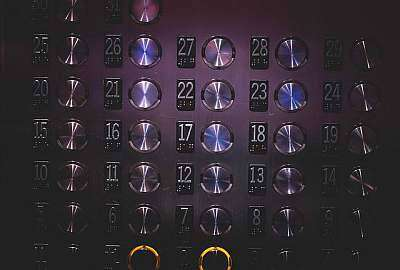# Percent to Fraction Calculator

Instructions: Use this calculator to convert a given percentage into a fraction, showing all the steps of the process. Please type in one percentage (for example, something like 34.54% or 6%) in the form below:Type one percent expression (Ex: 53.45%, or .4562%)

## Percent to Fraction Conversion

Often times, you will be interested in different types of conversions when dealing with fraction, decimals and percents. Indeed, there is a natural connection between fractions and percentages.

For example, a fraction like a percentage of 25% is often understood as a "one quarter", which is obviously represented by the fraction "1/4". Now, there is obviously not just one fraction associated to 25%, as all the same as 1/4, we can write 25/100.

### How do you convert a percent to fraction

The process involves two parts: one part is to convert the percent to a decimal, and then to convert the decimal into a fraction. For the first part, we use the formula

$D = \displaystyle \frac{P}{100}$

This formula shows how to get a decimal from the percent $$P$$, which is the number associated to the percent (without the % sign)

### Steps for the conversion of a percent to fraction

1. Identify the percentage you want to convert, and call it $$P$$, after you remove the "%" sign
2. Compute the associated decimal $$D$$, using the formula $$D = \displaystyle \frac{P}{100}$$
3. Find the number of digits in the decimal part and call it $$k$$
4. The numerator of the fraction you are looking for is 10^k * D, and the denominator is 10^k### Examples: Converting percents to fractions

What is 0.38% as a fraction?

Solution: Following the steps outlined, first we identify the given percentage, which in this case is $$P = 0.38\%$$. Removing the "%" sign leads to the number $$P = 0.38$$. Then, we divide this number by 100:

$D = \displaystyle \frac{P}{100} = \displaystyle \frac{0.38}{100} = 0.0038$

So then, 0.38% corresponds to 0.0038. Now we convert it to a fraction. The number of digits is $$k =4$$, so we multiply 0.0038 by 10^4 = 10,000, as we get the fraction $$\displaystyle \frac{38}{10000}$$, which can be further simplified to $$\displaystyle \frac{19}{5000}$$

### Useful calculators for decimals, fractions and percentages

If you are interest in the other way around, you can use this fraction to percent calculator, if instead you are provided with a fraction.

Very commonly used is this decimal to percent calculator, which allows you to convert between decimal and percentages.